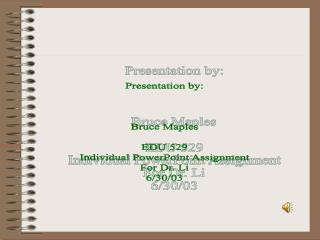DownloadDownload PresentationPresentation by: Bruce Maples EDU 529 Individual PowerPoint Assignment For Dr. Li 6/30/03

# Presentation by: Bruce Maples EDU 529 Individual PowerPoint Assignment For Dr. Li 6/30/03

Télécharger la présentation## Presentation by: Bruce Maples EDU 529 Individual PowerPoint Assignment For Dr. Li 6/30/03

- - - - - - - - - - - - - - - - - - - - - - - - - - - E N D - - - - - - - - - - - - - - - - - - - - - - - - - - -
##### Presentation Transcript

1. Presentation by: Bruce Maples EDU 529 Individual PowerPoint Assignment For Dr. Li 6/30/03

2. FIVE BASIC WAYS TO CLASSIFY CHEMICAL REACTIONS: • Synthesis • Decomposition • Single-Replacement • Double-Replacement • Combustion

3. Synthesis Reactions: • A synthesis reaction generally follows this equation: • X + Y-->XY • In this equation, X and Y can be compounds or elements. The result XY is a compound.

4. Examples of Synthesis Reactions: • 2Ba + O2 -->2BaO • 4Cu+ + O2 -->2Cu2O • 2Cu++ + O2 -->2CuO • 2H2 + O2 -->2H2O • C + O2 -->CO2 • 2Na + Cl2 -->2NaCl2

5. Decomposition Reactions: • In a decomposition reaction, an element or compound is broken down into two simpler substances. The general formula of a decomposition reaction is: • XY --> X + Y • Decomposition reactions are the opposite of synthesis reactions.

6. Examples of Decomposition Reactions: • 2HgO --> 2Hg + O2 • 2H2O --> 2H2 + O2 • CaCO3 --> CaO + CO2 • Cu(OH)2 --> CuO + H2O • 2KClO3 --> 2KCl + 3O2H2SO4 --> SO3 + H2O

7. Single Replacement Reactions: • In this type of reaction, one element replaces a similar element in a compound. There are two general equations for single-replacement reactions. They are: • A + XY --> AY + X • A + XY --> XA + Y • In this equation, A, X, and Y are the elements and XY, AY, and XA are the compounds.

8. Examples of Single Replacement Reactions: • 2Al + 3Fe(NO3)2 --> 3Fe + 2Al(NO3)3 • 2Na + 2H2O --> 2NaOH + H2 • 3Fe + 4H2O --> Fe3O4 + 4H2 • Mg + 2HCl --> MgCl2 + H2 • Cl2 + 2KBr --> 2KCl + Br2

9. Double-Replacement Reactions: • In a double-replacement reaction, the two compounds exchange places in a solution to form two new compounds. The general equation of a double-replacement reaction is: • AB + XY --> AY + BX

10. Examples of Double-Replacement Reactions: • Pb(NO3)2 + 2KI --> PbI2 + 2KNO3 • FeS + 2HCl --> H2S + FeCl2 • HCl + NaOH --> NaCl + H2O

11. Combustion Reactions: • When a substance combines with oxygen releasing a large amount of energy in the form of light and heat, it is a combustion reaction.

12. Examples of Combustion Reactions: • An example of a combustion reaction is: • C3H8 + 5O2 --> 3CO2 + 4H2O

13. Presented by Bruce Maples EDU 529 Individual PowerPoint Presentatio For Dr. Sha Li 6/30/03